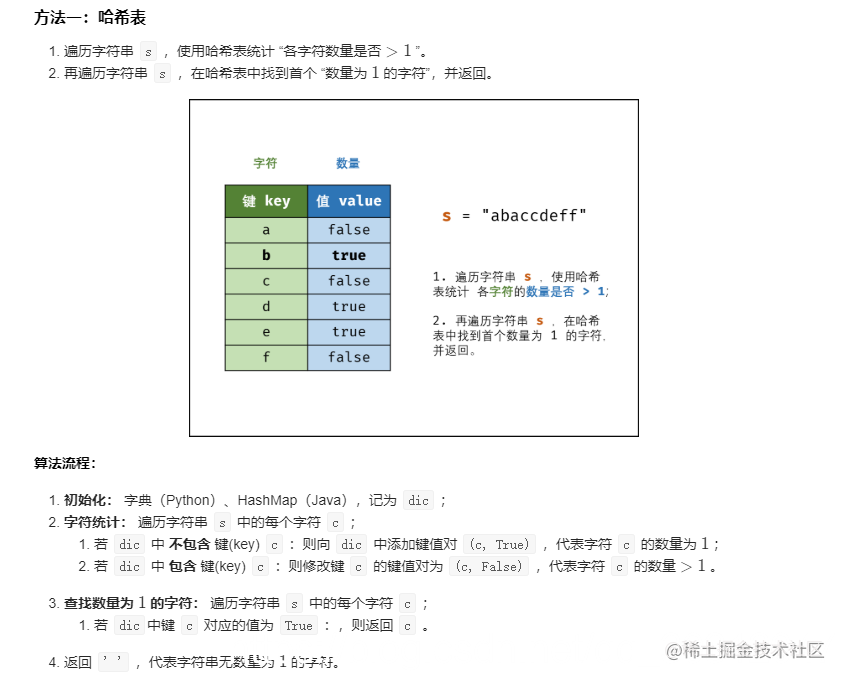# 剑指 Offer 50. 第一个只出现一次的字符

s = “abaccdeff”

s = “”

0 <= s 的长度 <= 50000## java

``````class Solution {
public char firstUniqChar(String s) {
// char b ='d';
// for(char a : s.toCharArray()){
//     System.out.print(s.count(a));
// }
// return b;

char []chars=s.toCharArray();   //将一个简单的字符串中的内容转化为char数组
HashMap<Character,Boolean> hm = new HashMap<>();
for(char c : chars){
hm.put(c,!hm.containsKey(c));

}

for(char c: chars){
//hm.keySet()代表所有键的集合,进行格式化输出
if (hm.get(c)) return c;
}
return ' ';
}
}